Open in App
Not now

# SciPy – Spatial Distance Matrix

• Last Updated : 17 Nov, 2021

A distance matrix contains the distances computed pairwise between the vectors of matrix/ matrices. scipy.spatial package provides us distance_matrix() method to compute the distance matrix. Generally matrices are in the form of 2-D array and the vectors of the matrix are matrix rows ( 1-D array).

```Syntax: scipy.spatial.distance_matrix(x, y, p=2)

Parameters:
x : (M, K) Matrix of M vectors, each of dimension K.
y : (N, K) Matrix of N vectors, each of dimension K.
p : float, 1 <= p <= infinity, defines which Minkowski p-norm to use.

Returns: (M, N) ndarray
/ matrix containing the distance from every vector in x to every vector in y.```

Note: the column dimensions of both x, and y matrices must be same.

We can use different values for p to apply different types of the distances to compute the distance matrix.

```p = 1, Manhattan Distance
p = 2, Euclidean Distance
p = ∞, Chebychev Distance```

Example 1.

We compute the distance matrix for two matrices x, and y. Both matrices have same dimension (3, 2).  So the distance matrix has dimension (3,3). Using p=2, the distances are calculated as Minkowski 2-norm (or Euclidean distance).

## Python3

 `# Python program to compute distance matrix` `# import important libraries``import` `numpy as np``from` `scipy.spatial ``import` `distance_matrix` `# Create the matrices``x ``=` `np.array([[``1``,``2``],[``2``,``1``],[``2``,``2``]])``y ``=` `np.array([[``5``,``0``],[``1``,``2``],[``2``,``0``]])` `# Display the matrices``print``(``"matrix x:\n"``, x)``print``(``"matrix y:\n"``, y)` `# compute the distance matrix``dist_mat ``=` `distance_matrix(x, y, p``=``2``)` `# display distance matrix``print``(``"Distance Matrix:\n"``, dist_mat)`

Output: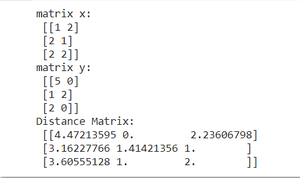distance matrix example 1

Example 2.

We compute the distance matrix for two matrices x, and y. Both matrices have different dimensions. Matrix x has dimension (3,2) and matrix y has dimension (5,2). So the distance matrix has dimension (3,5).

## Python3

 `# Python program to compute distance matrix` `# import important libraries``import` `numpy as np``from` `scipy.spatial ``import` `distance_matrix` `# Create the matrices``x ``=` `np.array([[``1``,``2``],[``2``,``1``],[``2``,``2``]])``y ``=` `np.array([[``0``,``0``],[``0``,``0``],[``1``,``1``],[``1``,``1``],[``1``,``2``]])` `# Display the matrices``print``(``"matrix x:\n"``, x)``print``(``"matrix y:\n"``, y)` `# compute the distance matrix``dist_mat ``=` `distance_matrix(x, y, p``=``2``)` `# display distance matrix``print``(``"Distance Matrix:\n"``, dist_mat)`

Output: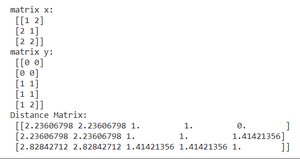distance matrix example 2

Example 3.

We compute the distance matrix using single matrix ( i.e. x). Matrix x has dimension (3,2). Same matrix x is given as parameter y. The distance matrix has dimension (3,3).

## Python3

 `# Python program to compute distance matrix` `# import important libraries``import` `numpy as np``from` `scipy.spatial ``import` `distance_matrix` `# Create the matrix``x ``=` `np.array([[``1``,``2``],[``2``,``1``],[``2``,``2``]])` `# Display the matrix``print``(``"matrix x:\n"``, x)` `# compute the distance matrix``dist_mat ``=` `distance_matrix(x, x, p``=``2``)` `# display distance matrix``print``(``"Distance Matrix:\n"``, dist_mat)`

output: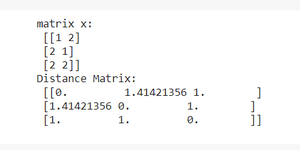distance matrix example 3

Note: Notice that the above distance matrix is a symmetric matrix. When both x, and y matrices are same, the distance matrix is a symmetric matrix.

Example 4.

We compute the distance matrix for two matrices x, and y. Both matrices have different dimensions. Matrix x has dimension (3,2) and matrix y has dimension (5,2). So the distance matrix has dimension (3,5). Using p=1, the distances are calculated as Minkowski 1-norm (or Manhattan Distance).

## Python3

 `# Python program to compute distance matrix` `# import important libraries``import` `numpy as np``from` `scipy.spatial ``import` `distance_matrix` `# Create the matrices``x ``=` `np.array([[``1``,``2``],[``2``,``1``],[``2``,``2``]])``y ``=` `np.array([[``5``,``0``],[``1``,``2``],[``2``,``0``]])` `# Display the matrices``print``(``"matrix x:\n"``, x)``print``(``"matrix y:\n"``, y)` `# compute the distance matrix``dist_mat ``=` `distance_matrix(x, y, p``=``1``)` `# display distance matrix``print``(``"Distance Matrix:\n"``, dist_mat)`

Output: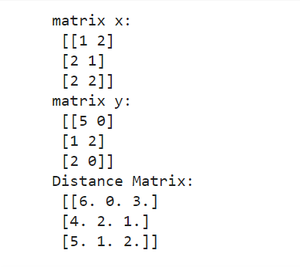distance matrix example 4

Example 5.

We compute the distance matrix for two matrices x, and y. Both matrices have dimension (2, 5). So the distance matrix has dimension (3,5). Using p=2, the distances are calculated as Minkowski 2-norm (or Euclidean Distance).

## Python3

 `# Python program to compute distance matrix` `# import important libraries``import` `numpy as np``from` `scipy.spatial ``import` `distance_matrix` `# Create the matrices``x ``=` `np.array([[``1``,``2``,``3``,``4``,``5``],[``2``,``1``,``0``,``3``,``4``]])``y ``=` `np.array([[``0``,``0``,``0``,``0``,``1``],[``1``,``1``,``1``,``1``,``2``]])` `# Display the matrices``print``(``"matrix x:\n"``, x)``print``(``"matrix y:\n"``, y)` `# compute the distance matrix``dist_mat ``=` `distance_matrix(x, y, p``=``2``)` `# display distance matrix``print``(``"Distance Matrix:\n"``, dist_mat)`

Output: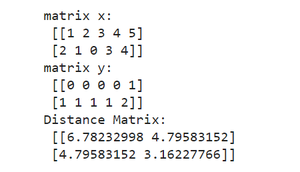distance matrix example 5

My Personal Notes arrow_drop_up